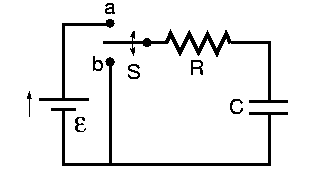# Problem: Which of the following statements are correct for the RC circuit shown? Select true or false for each statement.You may assume that when the switch is in position b for a long time, the capacitor is fully discharged (Q = 0).A. The longer S is kept in the position a, the lower is the energy stored in C.B. Right after S is moved to the position a, following a long time in the position b, the current through R is zero.C. Right after S is moved to the position a, following a long time in the position b, the current through R is ε/R.D. The longer S is kept in the position a, the higher is the energy stored in C.E. If S is in the position a for a long time, the current through R approaches zero.F. If S is in the position a for a long time, the charge on C approaches zero.

###### FREE Expert Solution

A.

The energy stored in a capacitor is given by:

$\overline{){\mathbf{U}}{\mathbf{=}}\frac{\mathbf{1}}{\mathbf{2}}{\mathbf{Q}}{\mathbf{V}}{\mathbf{=}}\frac{\mathbf{1}}{\mathbf{2}}{\mathbf{C}}{{\mathbf{V}}}^{{\mathbf{2}}}}$

82% (322 ratings)###### Problem Details

Which of the following statements are correct for the RC circuit shown? Select true or false for each statement.
You may assume that when the switch is in position b for a long time, the capacitor is fully discharged (Q = 0).A. The longer S is kept in the position a, the lower is the energy stored in C.
B. Right after S is moved to the position a, following a long time in the position b, the current through R is zero.
C. Right after S is moved to the position a, following a long time in the position b, the current through R is ε/R.
D. The longer S is kept in the position a, the higher is the energy stored in C.
E. If S is in the position a for a long time, the current through R approaches zero.
F. If S is in the position a for a long time, the charge on C approaches zero.